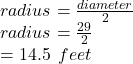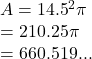## A circle has a diameter of 29 feet. What is the area? * 650.5 square feet 655.5 square feet O 660.5 square feet O 66

Question

A circle has a diameter of 29 feet. What is the area? *
650.5 square feet
655.5 square feet
O 660.5 square feet
O 665.5 square feet

in progress 0
7 months 2021-08-14T06:01:45+00:00 1 Answers 0 views 0

## 660.5 square feet

Step-by-step explanation:

Area of a circle = πr²

where r is the radius

From the question

diameter = 29 feet

To find the radius given the diameter we use the formulaSo the area of the circle isWe have the final answer as

### 660.5 square feet

Hope this helps you